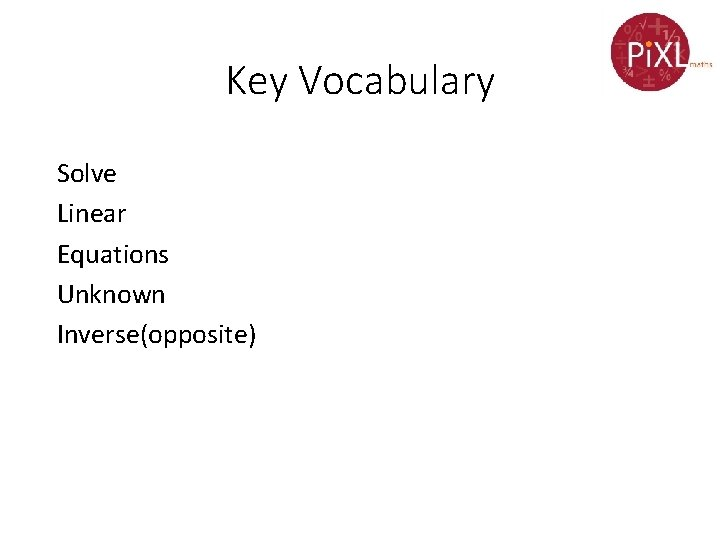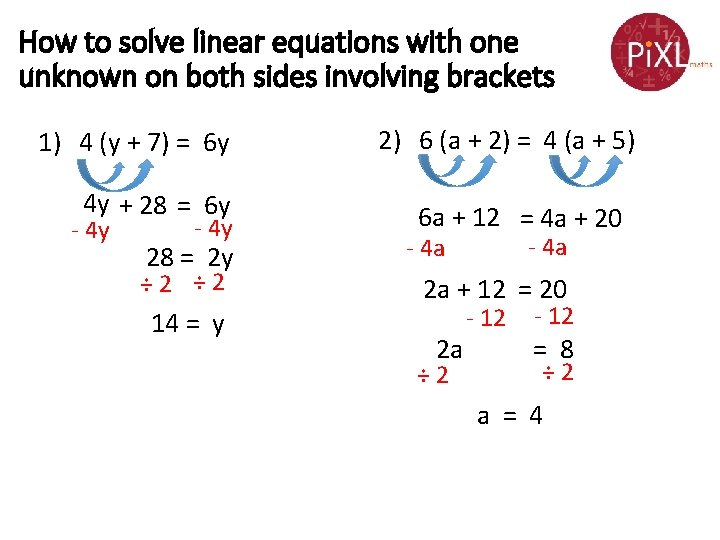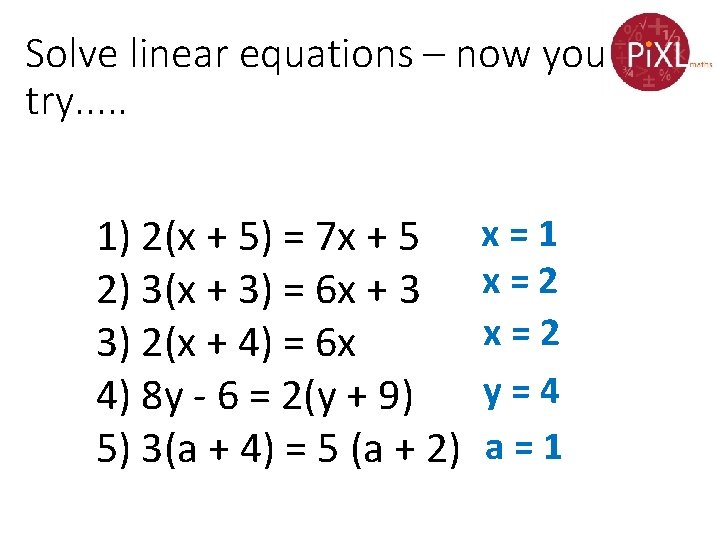# Grade C Linear Equations Solve linear equations with

• Slides: 8Grade C Linear Equations Solve linear equations with one unknown on both sides and those involving brackets If you have any questions regarding these resources or come across any errors, please contact [email protected] org. ukKey Vocabulary Solve Linear Equations Unknown Inverse(opposite)How to solve linear equations with one unknown on both sides 1) 11 x = 9 x + 8 - 9 x 2) 5 x + 16 = 9 x - 5 x 2 x = 8 16 = 4 x x=4 4=x ÷ 2 ÷ 4How to solve linear equations with one unknown on both sides 3) 10 x + 6 = 6 x + 26 - 6 x 4 x + 6 = 26 -6 4 x = 20 ÷ 4 x=5 ÷ 4 -6 4) 5 x - 6 = 7 x - 10 - 5 x - 6 = 2 x - 10 + 10 4 = 2 x ÷ 2 2=xSolve linear equations – now you try. . . 1) 2) 3) 4) 5) 5 x = 3 x + 10 x = 5 6) 2 x + 10 = 7 x + 5 x = 1 6 x = 3 x + 9 x = 3 7) 3 x - 5 = x + 10 x = 7. 5 3 x + 12 = 6 x x = 4 8) 9 x - 12 = 5 x - 4 x = 2 2 x – 20 = 7 x x = -4 9) 6 x + 6 = 36 – 4 x x = 3 5 x + 10 = 3 x + 16 x = 3 10) 10 – 3 x = 16 – 5 x x=3How to solve linear equations with one unknown on both sides involving brackets 1) 4 (y + 7) = 6 y 4 y + 28 = 6 y - 4 y 28 = 2 y ÷ 2 14 = y 2) 6 (a + 2) = 4 (a + 5) 6 a + 12 = 4 a + 20 - 4 a 2 a + 12 = 20 2 a ÷ 2 - 12 = 8 ÷ 2 a = 4Solve linear equations – now you try. . . 1) 2(x + 5) = 7 x + 5 2) 3(x + 3) = 6 x + 3 3) 2(x + 4) = 6 x 4) 8 y - 6 = 2(y + 9) 5) 3(a + 4) = 5 (a + 2) x=1 x=2 y=4 a=1More Practice Solving Linear Equations a) 3(x – 3) = x + 1 b) 2(x + 6) = 6 x c) 2(3 + x) = 8 x – 3 d) 2 (a + 5) = 6 ( a – 5) e) 5 (y – 6) = 2 ( 6 + y) f) 4 b = 6(b – 2) x=5 x=3 x = 1. 5 a = 10 y = 14 b=6